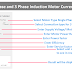Here, in this Induction Motor Current Calculator, you can calculate Rated or Running Load Current(RLA), Full Load Current(FLA), Locked Rotor Current(LRA) or Starting Current, Voltage Dip During Starting, etc. Here, you can calculate for both 3 Phase and Single Phase Induction Motors.

Induction Motor Current Calculator

## Induction Motor Current Calculator

Results:

You Must Know about these terms while calculating motor current,

2. Rated Current, also known as Running Load Amps(RLA)

3. Starting Current, also known as Locked Rotor Amps(LRA)

### How to Use This Calculator?

To use the above "Induction Motor Current Calculator," follow the below steps:

1. Select Motor Type: Choose between "Single Phase" or "Three Phase" by clicking the respective radio button.

2. Select Connection Type for Three-Phase Motor: If you select the three-phase motor, further options will be displayed. And then you have to choose between "Delta Connected" or "Star Connected" by clicking the appropriate radio button.

3. Enter Supply Voltage: Input the supply voltage or motor voltage value in the specified input box. You can enter the value in Volts or Kilovolts by choosing the appropriate option from the dropdown.

4. Enter Motor Power Rating: Enter the power rating of the motor in the input box. You can enter the value in Watts, Kilowatts, or Horsepower by choosing the appropriate option from the dropdown.

5. Enter Power Factor: Input the power factor of the motor in the provided input box. The power factor is a unitless value typically between 0 and 1.

6. Enter Motor Efficiency: Input the motor efficiency percentage in the provided input box. The efficiency value should be between 0 and 100. If you don't know them you can put 85% by default.

7. Enter Service Factor: If applicable, input the service factor of the motor. This value is usually recommended to be 1.15 but can vary depending on the specific motor application.

8. Select Output Current Unit: Choose the unit for the output current that you want to see in the result.  Select one of the options from the dropdown: Amp, Milliamp, or Kiloamp.

9. Calculate: Click the "Calculate" button to obtain the results. The calculated results will be displayed in the "Results:" section.

### Formula

Here are the formulas, the calculator uses to calculate the current of the induction motor.

Rated Current (RLA) Calculation formula for Single Phase Motor:

RLA = (Power Rating in Watts) / (Voltage in Volts * Efficiency * Power Factor)

Rated Current (RLA) Calculation formula for Three Phase Motor (Star Connected):

RLA = (Power Rating in Watts) / (3 * Voltage in Volts * Efficiency * Power Factor)

Rated Current (RLA) Calculation formula for Three Phase Motor (Delta Connected):

RLA = (Power Rating in Watts) / (1.732 * Voltage in Volts * Efficiency * Power Factor)

Full Load Current (FLA) Calculation Formula:

FLA = RLA * Service Factor

Expected Starting Current Calculation Formula:

Starting Current = FLA * 6

Voltage Drop during Starting Calculation Formula:

Voltage Drop = Impedance * Starting Current

Expected Voltage Dip During Starting:

Starting Voltage = Supply Voltage - Voltage Drop

Where:

RLA: Rated Load Amps (the current drawn by the motor at rated load or running conditions)
FLA: Full Load Amps (the current drawn by the motor at full load conditions)
Starting Current: (the current drawn by the motor during starting)
Efficiency: Motor efficiency (expressed as a decimal)
Power Factor: Motor power factor (expressed as a decimal)
Service Factor: Motor service factor
Impedance: Impedance of the motor, calculated as (Voltage^2 * Power Factor) / Power Rating

Thank you for visiting the Website. Keep visiting for more Updates.

Induction Motor Current Calculator (Rated, Starting, Full Load)Reviewed by Author on August 04, 2023 Rating: 5